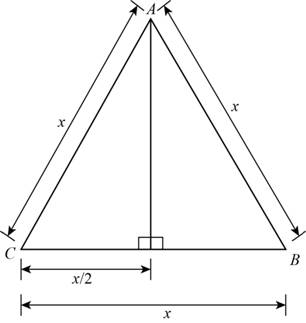# The function that models area of equilateral triangle in terms of its length x of one of its sides.### Precalculus: Mathematics for Calcu...

6th Edition
Stewart + 5 others
Publisher: Cengage Learning
ISBN: 9780840068071### Precalculus: Mathematics for Calcu...

6th Edition
Stewart + 5 others
Publisher: Cengage Learning
ISBN: 9780840068071

#### Solutions

Chapter 2, Problem 7P
To determine

## To find: The function that models area of equilateral triangle in terms of its length x of one of its sides.

Expert Solution

The function that models area of given equilateral triangle is 34x2 .

### Explanation of Solution

Draw the figure of equilateral triangle as shown below.Figure (1)

Area of triangle is,

A=12(base)(height)

The length of side of equilateral triangle is x .

In Figure (1) AD is median, it bisects CB so CD is x2 .

Use Pythagorean theorem in triangle ACD shown in Figure (1).

Substitute x for AC , x2 for CD in above equation.

Subtract x24 from both sides of above equation.

Take square root of above equation and switch sides.

Summarize the all the information as shown in table below.

 In Words In Algebra Base x Height 34x

Use the information in the table and model the function.

A=12(base)(height)=12(x)(34x)=12(x)(32x)=34x2

Thus, the function that models area of equilateral triangle is A(x)=34x2 .

### Have a homework question?

Subscribe to bartleby learn! Ask subject matter experts 30 homework questions each month. Plus, you’ll have access to millions of step-by-step textbook answers!